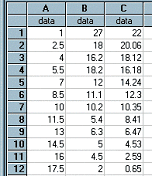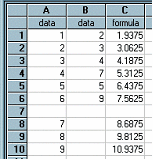﻿ Predict(column1, column2, regtype) / PredictY()

# Predict(column1, column2, regtype) / PredictY()

Top  Previous  Next
 These two functions are equivalent.   column1 contains the independent variable's data (usually x values) column2 contains the dependent variable's data (usually y values) regtype is the regression model    1        linear regression 2        quadratic regression 3        power regression 4        exponential regression 5        logarithmic regression 6        median line 7        reciprocal regression Please note that it is not necessary to remember or use these codes.   Predict calculates the predicted value of y for the x in column1 using the regtype regression model.   All three parameters are optional.  If they are not included, the current column one, column two and regression type as shown in the Statistics View are used.  On most occasions you would simply type Predict( )   In the first example, column C has been generated using Predict( ).  We did not use any parameters because the Statistics View was showing Linear Regression Calculations for A and B.  We could have typed Predict(A,B,1) and got the same result regardless of the Statistics View.  Column C fits the equation y = 23.39 – 1.29x.Predict can be used to predict y values for x values that are not in the data set.  Simply add extra x values with no corresponding y values.  Predict will correctly calculate a predicted y value.  If you include a y value that corresponds with an x value, the pair will automatically be included in the data set.  The second example shows predicted y scores for the x values of 7, 8 and 9.Note:  If you type decimals using a comma (eg 3,2) you should enter this formula as Predict(A; B; 1)  - using the ; as a separator